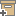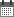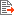ניהול
קהילה:
אסיף מאגר המחקר החקלאי
A theoretical study of the estimation of the correlation scale in spatially variable fields: 2. Nonstationary fieldsBack to searchPrevious item
Next item
Year:
1987
Source of publication :
Water Resources Research
Authors :
רוסו, דוד
;
.
Volume :
23
Co-Authors:
Russo, D.
Jury, W.A.
Facilitators :
From page:
1269
To page:
1279
(
Total pages:
11
)
Abstract:
A nonstationary field may be viewed as a process composed of two components, a stochastic component characterized by a relatively small‐scale, high‐frequency variation (HFV), and a deterministic (drift) component characterized by a relatively large‐scale, low‐frequency variation (LFV). In this case, the estimation of the variogram or the covariance function and its associated correlation scale requires the prior estimation of the drift. In this study, the effects of the type of drift function, the size of the integral scale, and the variances associated with the two components on the estimation of the correlation scale were analyzed using a theoretically generated master population of points representing the nonstationary field. A set of nonstationary fields was generated by superimposing different drift functions with a given variance σL 2 on stationary fields with a given integral scale and variance σH 2. Results showed that the shape of the estimated variograms and their associated estimated correlation scales, the fractal dimensions, and the estimates of the covariance function provided by the parametric estimation procedures were affected by the type of the imposed drift function, by the integral scale of the stochastic component, and by the ratio between the variances of the two components. Both the maximum likelihood (ML) and the restricted maximum likelihood (RML) estimation procedures may fail to correctly identify and separate the two components when their respective variances are of the same order of magnitude and when the correlation scale or spatial extent of the stochastic component is small compared with the typical size of the field domain. In these situations, the fractal dimension analysis also may not indicate the dominance of the LFV over the HFV even when σL 2 ≃ 2σH 2. The model validation tests also may fail to indicate inconsistency between the estimates of the parameters of the structural model and the data, when σL 2 ≃ σH 2. In general, the ML and the RML estimation procedures overestimated the integral scale J and the variance C(O) of the underlying process, whereas the iterative generalized least squares and the ordinary least squares procedures coupled with the minimum mean‐square deviation (MSD) criterion for variogram model fitting underestimated the value of the integral scale. However, results of model validation tests suggested that these estimates were accurate and consistent with the data and almost indistinguishable in terms of the values of the Akaike Information Criterion. Consequently, different sets of Ĵ and Ĉ(0) values may adequately describe a given realization of the nonstationary field to the same level of precision. Careful examination of the estimated variograms over the entire set of lag classes, application of fractal dimension analysis, and a validation test of the fitted variogram models are necessary steps for drift detection. Quantification of the drift, however, may not be achieved in certain situations. Copyright 1987 by the American Geophysical Union.
Note:
Related Files :
ESTIMATED VARIOGRAMS
Mathematical models
MATHEMATICAL TECHNIQUES
NONSTATIONARY FIELDS
Soils
עוד תגיות
תוכן קשור
More details
DOI :
10.1029/WR023i007p01269
Article number:
Affiliations:
Database:
סקופוס
Publication Type:
מאמר
;
.
Language:
אנגלית
Editors' remarks:
ID:
20842
Last updated date:
02/03/2022 17:27
Creation date:
16/04/2018 23:39Scientific Publication
A theoretical study of the estimation of the correlation scale in spatially variable fields: 2. Nonstationary fields
23
Russo, D.
Jury, W.A.
A theoretical study of the estimation of the correlation scale in spatially variable fields: 2. Nonstationary fields
A nonstationary field may be viewed as a process composed of two components, a stochastic component characterized by a relatively small‐scale, high‐frequency variation (HFV), and a deterministic (drift) component characterized by a relatively large‐scale, low‐frequency variation (LFV). In this case, the estimation of the variogram or the covariance function and its associated correlation scale requires the prior estimation of the drift. In this study, the effects of the type of drift function, the size of the integral scale, and the variances associated with the two components on the estimation of the correlation scale were analyzed using a theoretically generated master population of points representing the nonstationary field. A set of nonstationary fields was generated by superimposing different drift functions with a given variance σL 2 on stationary fields with a given integral scale and variance σH 2. Results showed that the shape of the estimated variograms and their associated estimated correlation scales, the fractal dimensions, and the estimates of the covariance function provided by the parametric estimation procedures were affected by the type of the imposed drift function, by the integral scale of the stochastic component, and by the ratio between the variances of the two components. Both the maximum likelihood (ML) and the restricted maximum likelihood (RML) estimation procedures may fail to correctly identify and separate the two components when their respective variances are of the same order of magnitude and when the correlation scale or spatial extent of the stochastic component is small compared with the typical size of the field domain. In these situations, the fractal dimension analysis also may not indicate the dominance of the LFV over the HFV even when σL 2 ≃ 2σH 2. The model validation tests also may fail to indicate inconsistency between the estimates of the parameters of the structural model and the data, when σL 2 ≃ σH 2. In general, the ML and the RML estimation procedures overestimated the integral scale J and the variance C(O) of the underlying process, whereas the iterative generalized least squares and the ordinary least squares procedures coupled with the minimum mean‐square deviation (MSD) criterion for variogram model fitting underestimated the value of the integral scale. However, results of model validation tests suggested that these estimates were accurate and consistent with the data and almost indistinguishable in terms of the values of the Akaike Information Criterion. Consequently, different sets of Ĵ and Ĉ(0) values may adequately describe a given realization of the nonstationary field to the same level of precision. Careful examination of the estimated variograms over the entire set of lag classes, application of fractal dimension analysis, and a validation test of the fitted variogram models are necessary steps for drift detection. Quantification of the drift, however, may not be achieved in certain situations. Copyright 1987 by the American Geophysical Union.
Scientific Publication
You may also be interested in